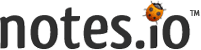Fast | Easy | Short

public class Cesar {
public static String encrypt (String plainText,int shift){
if (shift>26){
shift=shift%26;}
else if(shift<0){
shift=(shift%26)+26;
}
String cipherText ="";
int length=plainText.length();
for(int i=0;i<length;i++){
char ch = plainText.charAt(i);
if(Character.isLetter(ch)){
if(Character.isLowerCase(ch)){
char c = (char)(ch+shift);
if (c>'z'){
cipherText += (char)(ch -(26-shift));
}
else cipherText +=c;
}
else if(Character.isUpperCase(ch)){
char c = (char) (ch+shift);
if (c>'Z'){
cipherText += (char) (ch -(26-shift));
}
else cipherText +=c;

}
}
else{cipherText +=ch;
}
}
return cipherText;
}
public static String decrypt (String plainText,int shift){
if (shift>26){
shift=shift%26;}
else if(shift<0){
shift=(shift%26)+26;
}
String cipherText ="";
int length=plainText.length();
for(int i=0;i<length;i++){
char ch = plainText.charAt(i);
if(Character.isLetter(ch)){
if(Character.isLowerCase(ch)){
char c = (char) (ch-shift);
if (c<'a'){
cipherText += (char) (ch +(26-shift));
}
else cipherText +=c;
}
else if(Character.isUpperCase(ch)){
char c = (char) (ch-shift);
if (c<'A'){
cipherText += (char) (ch +(26-shift));
}
else cipherText +=c;

}
}
else{cipherText +=ch;
}
}
return cipherText;
}

public static void main(String[] args) {
String text ="This is some message";
String cipher = encrypt (text,2);
System.out.println(cipher);
String decrypt= decrypt(cipher,2);
System.out.println(decrypt);
}

}Notes.io is a web-based application for taking notes. You can take your notes and share with others by providing the shorten url to a friend.

Fast: Notes.io is built for speed and performance. You can take notes quickly and browse your archive.

Easy: Notes.io doesn’t require installation. Just write and share shorten link!

Short: Notes.io’s url just 8 character. You’ll get shorten link of your note when you want to share. (Ex: notes.io/q

Contact: hello@notes.io# Long File

For written notes was greater than 18KB Unable to shorten.